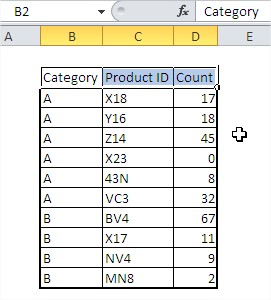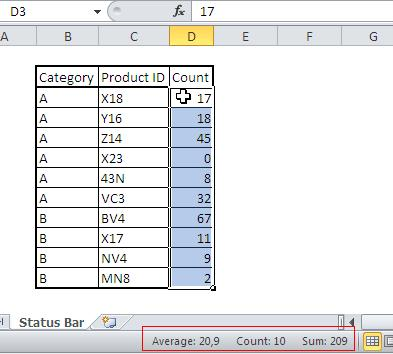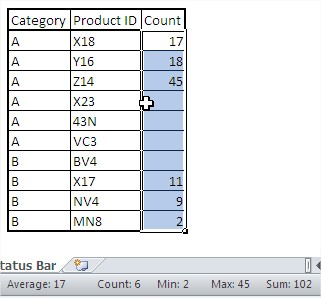### Status Bar in Excel

In this lesson you will learn what is and how to use Status Bar.

The table in this example are the sales volumes of products that belong to two categories.You want to quickly find out what is the total or average sales and how much has been sold? You could use the SUM, and AVERAGE, COUNT to obtain this information, but much faster will check the data and look at the status bar (at the bottom of the screen) where all this information is given (the numerator gives number of selected items and ignores empty cells).Average, Count and Total are the standard settings of the status bar, but you can change them by right-clicking on the status bar. At this moment you are only interested in the options marked in the figure below a red frame.Let’s add a Minimum and Maximum. Now five additional information will be given the status bar.Note that all calculations are made without the blank cells, so if you assume 4 products failed to sell at all and delete their data, the average will be calculated only for the 6 products and will be 17, and the sale will be given a minimum value of 2. Not zero!To avoid such problems for products that failed to sell, enter zero. In the figure below the correct values are calculated, the average is 12 and minimum 0.## Template

```Further reading:
```Further reading: